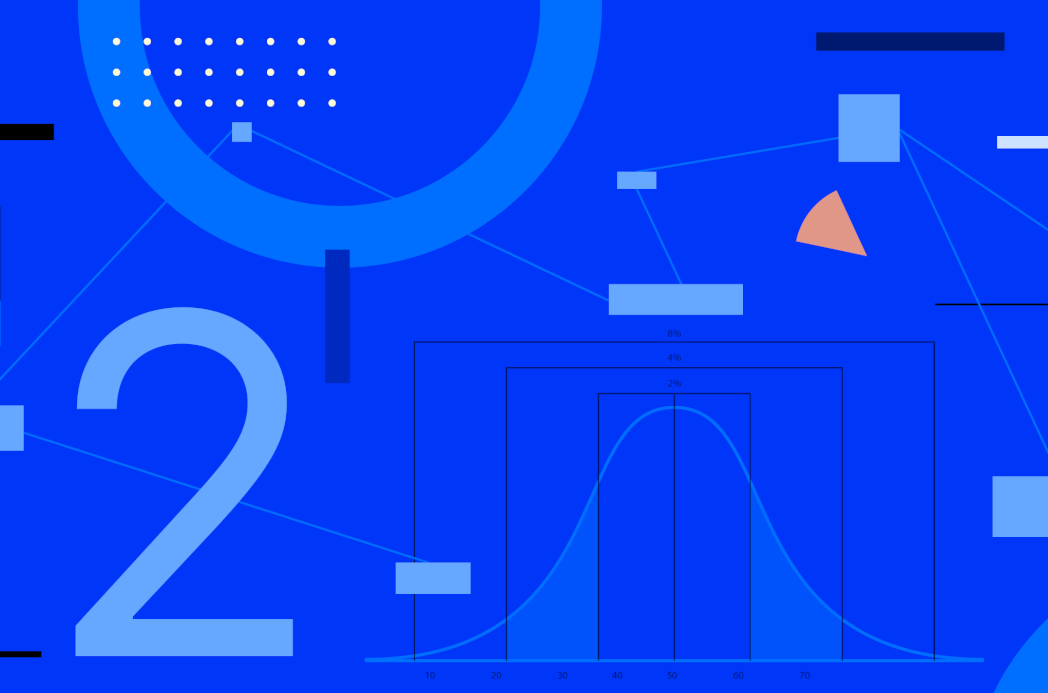AI / Machine Learning
-
April 20, 2020

# Understanding Basic Statistics for Machine Learning Models - Part 2In this article, you will find basic information about distributions. It is expected that you have some knowledge about random variables and probability concepts such as variance, covariance, and expected value. You can find that information on Understanding Basic Statistics for Machine Learning Models - Part 1

# What is a distribution?

A probability distribution: is a summary of probabilities for the values of a random variable.

Measurements: The distribution also has general properties that can be measured. Important properties of a probability distribution are: expected value, variance, skewness and kurtosis.

The probability for a discrete random variable can be summarized with a discrete probability distribution. In the same way, the summary for a continuous random variable is called continuous probability distribution.

Discrete data involves a finite group of possible values, while continuous data can have infinitive values in a time interval.

## Discrete distributions

Bernoulli:  distribution for a binary variable, represents the probability of a single experiment with 2 possible outcomes (probability p and 1-p).

Binomial: represents the number of successes in n Bernoulli trials. Thus, the experiments are independent, where each one has 2 possible outcomes.

Negative binomial: represents the number of successes (r) in a sequence of i.i.d. Bernoulli trials with probability p before a specified number of failures occurs.

Poisson distribution: models the number of events produced by a random process during a fixed interval of time or space. Lambda is the rate of events or arrivals within disjoint intervals.

## Continuous distributions

### Normal

A normal distribution is symmetric about the mean, where data near the mean is more frequent in occurrence than data far from the mean. It has a bell shape.
N( μ , σ )

Empirical rule:

The empirical rule establishes:

• Approximately 68% of the data falls within one standard deviation of the mean
• Approximately 95% of the data falls within two standard deviations of the mean
• Approximately 99.7% of the data falls within three standard deviations of the mean

### Truncated normal

A truncated normal distribution is a variation of the normal distribution, but the random variables are bound from either below or above, or both.

### Uniform

A uniform distribution describes an experiment with arbitrary outcomes in a certain bounds determined by  a and b, minimum and maximum values for the interval. The interval can be either open (a,b) or closed [a,b].

### Exponential

An exponential distribution expresses the probability distribution for time between intervals in a Poisson point process.

### Triangular

A triangular distribution is limited by a lower, upper limits and the mode value.

### Bimodal distribution

A bimodal distribution involves 2 different models.

### Multimodality

A multimodal distribution involves more than 2 models ( data comes from more than 2 groups).

### Skewness

The skewness measures lack of symmetry. A distribution, or data set, is symmetric if it looks the same to the left and right of the center point. Thus, it can be described as the measure of the distortion from a normal distribution.

A distribution that has negative skewness commonly indicates that the tail is on the left side, and in the same way positive skew indicates that the tail is on the right.

### Kurtosis

Kurtosis measures extreme values in either left or right tail. Measures whether the data is heavy-tailed in comparison with a normal distribution. When there is a large kurtosis, the tail exceeds the tail of the normal distribution, and It means that the dataset has outliers. Whereas data sets that have low kurtosis tend to have light tails, or lack of outliers.

### Quantiles

The quantiles are equal portions that divide a distribution. The image shows the 4 quantiles for a normal distribution. When the divisions correspond to 25%, 50% and 75% of the total distribution are called quartiles. The inter-quartile range is the difference between Q3 and Q1, and the 2nd quartile is the median.

### Confidence interval

A confidence interval is the level of certainty that the true parameter is in the proposed range. The confidence interval represents the probability of containing the true interval. In other words, it represents the proportion of intervals that contain the true value of the statistical parameter. The graph represents a confidence interval for 95%. The level of confidence is 95% and the likelihood that the true population parameter lies outside is  α, in this case  α = 0.5 = 1 - 0.95.